# Learn Different Ways to Use the IFS and VLOOKUP Nested Function

Jul 15, 2023Do you want an Excel formula that can save time and simplify worksheet management?

Using nested functions such as IFS and VLOOKUP might be the answer.

Combining these two powerful functions allows you to use their strengths to achieve even more complex data manipulation. Moreover, you don't have to input information from scratch manually.

In this blog post, we'll break down these two functions and how they work together to make your workflow more efficient.

Read on as we cover the following:

• The IF and VLOOKUP Functions

• Use VLOOKUP to Match a Specific Value

• Use the IF and VLOOKUP Nested Formula to Lookup Based on Two Values

• Matching Lookup Returns with Another Cell

• Use Nested Function with IF & VLOOKUP to Lookup Values from a Shorter List

• Use of IF and VLOOKUP Nested Function to Perform Different Calculations

• Final Thoughts on the IFS and VLOOKUP Nested Function

• Frequently Asked Questions on the IFS and VLOOKUP Nested Function## The IF and VLOOKUP Functions

The IF and VLOOKUP functions are some of the most used functions in Microsoft Excel for different purposes.

You can use the IF statement to set a condition, and the VLOOKUP is a reference function to look for a particular value within a range. These formulas allow specific values to search for within any dataset.

## Use IF and VLOOKUP to Match a Specific Value

In our example, the dataset includes the products' ID, name, unit price, quantity, total amount, and delivery date.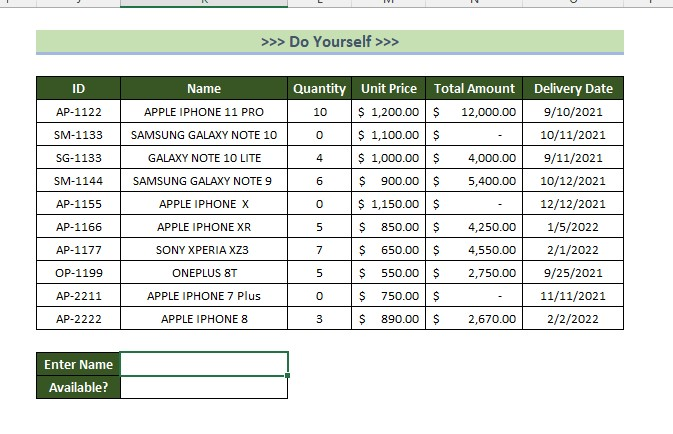To check the availability of products using the IF and VLOOKUP formulas, we will enter the product name, and the formula will search for its availability. Follow the steps below to do this:

1. Select a cell.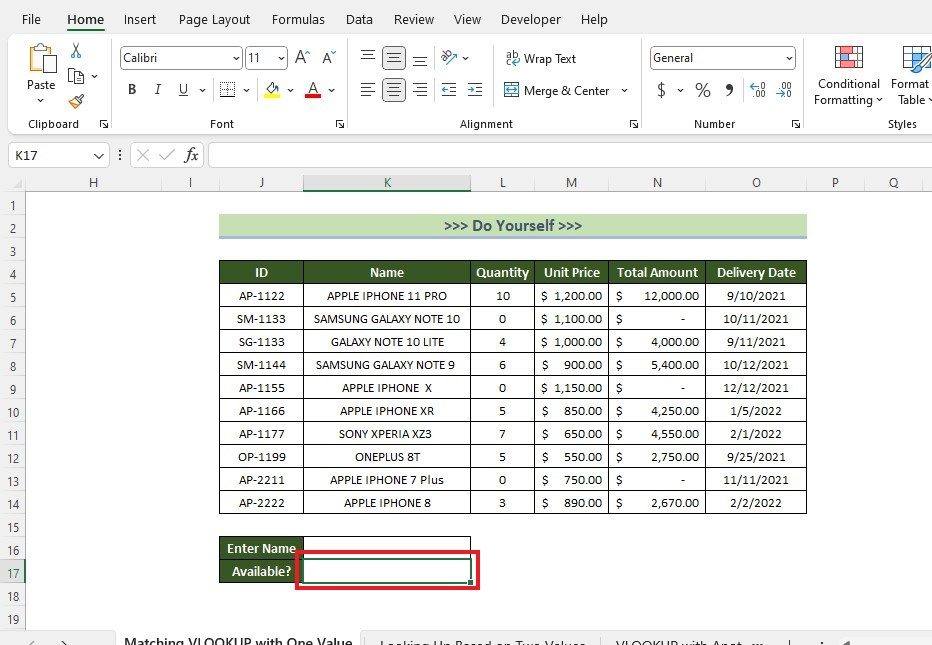1. Enter the IF and VLOOKUP formulas.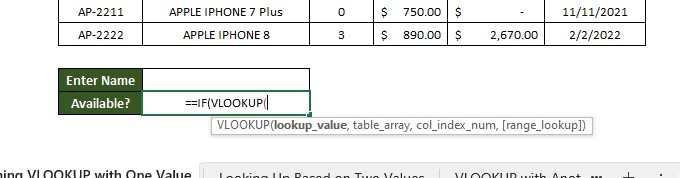1. Complete the IF and VLOOKUP formula.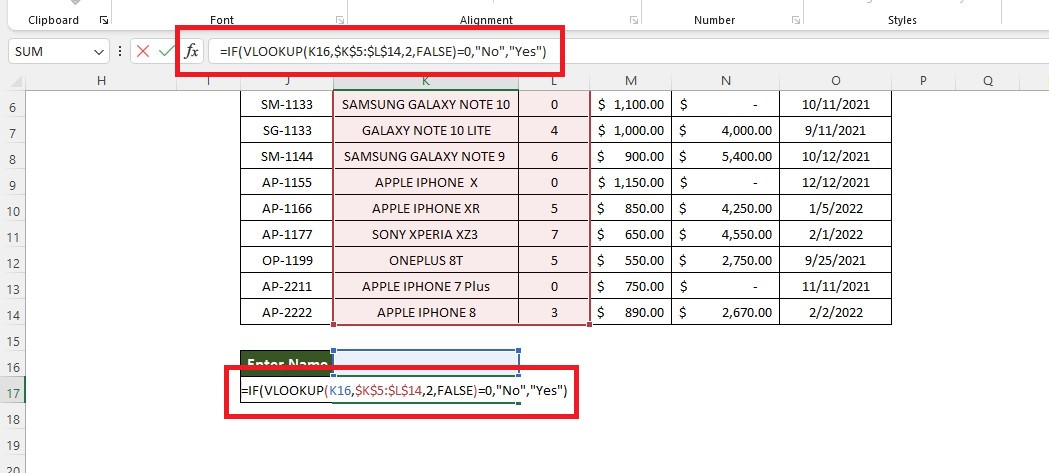1. Press the Enter key and type the lookup value you want ahead of the IF and VLOOKUP formula cell.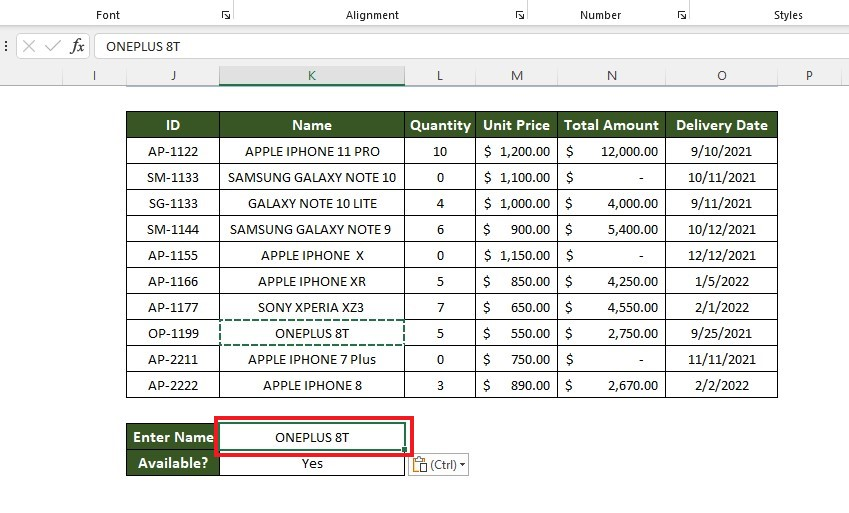Formula breakdown:

• In the VLOOKUP function, K16 is the cell holding the search keyword. Then, we'll include the range where we will search the entered data.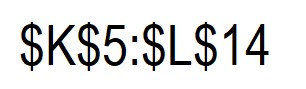• The number "2" is for looking for match criteria values in the second column of our lookup range. Meanwhile, FALSE will define the exact match.

• So, the VLOOKUP formula will return the value of the Quantity column for cell C16.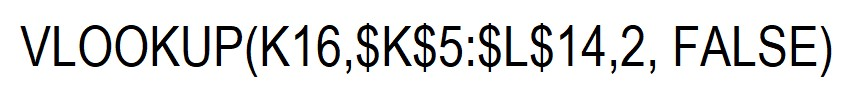• The IF function checks whether the output of the VLOOKUP function is 0 or something else. Moreover, the IF function will return Yes or No as the final output, depending on the result.

## Use the IF and VLOOKUP Nested Formula to Lookup Based on Two Values

We will use the nested functions IF and VLOOKUP to search for elements or products based on two values. Each product in the dataset has two market prices.

The two values required for the search are the product id and market number.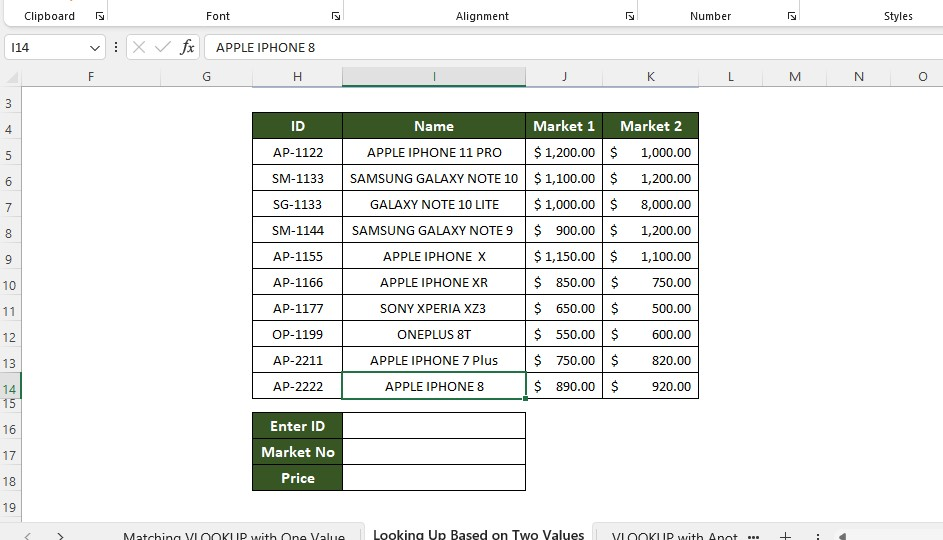We need to determine the price of the product using the given values. Follow the steps below to lookup based on multiple values:

1. Enter the following formula in cell I18.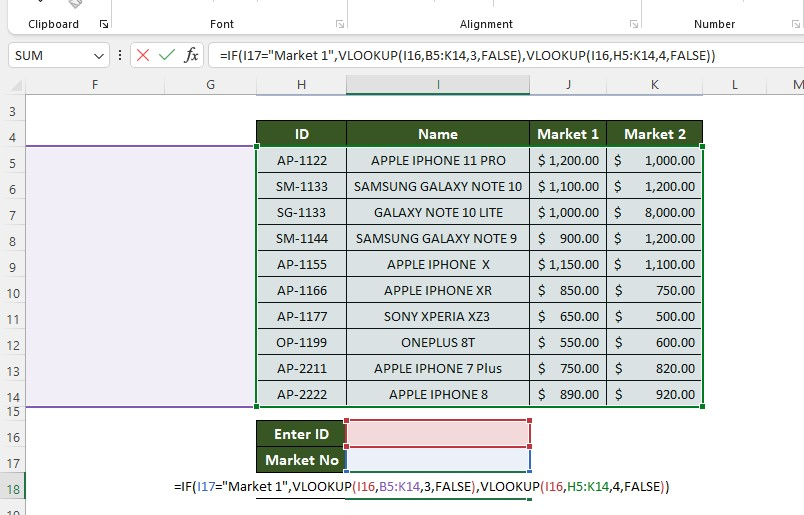1. Type the ID and market number to trigger the formula.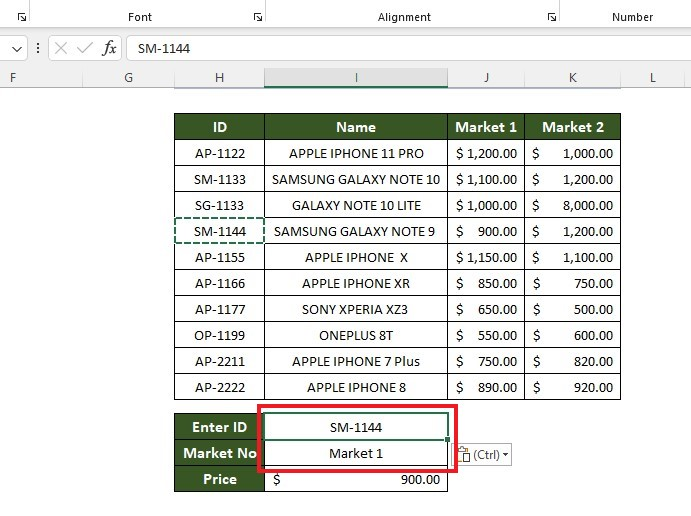Formula breakdown:

• In the IF function, I17= "Market 1" is the logical condition. It checks whether the entered "Market No" is one or not.

• If the "Market No" is 1, the price will be extracted from the Market 1 column using the first VLOOKUP formula part.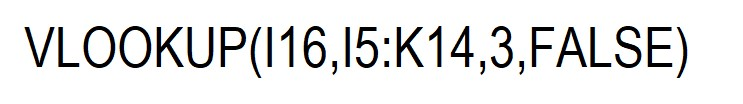• Otherwise, it will extract the price from the Market 2 column using the VLOOKUP formula in this subformula.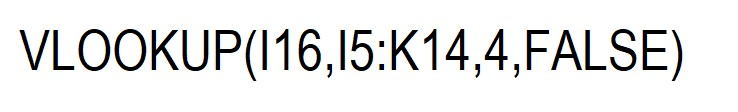## Matching Lookup Returns with Another Cell

In this method, we will to determine the highest price from the given data.

Then, we will compare this highest price with the entered data to see if there is a match.

1. Enter the MAX function.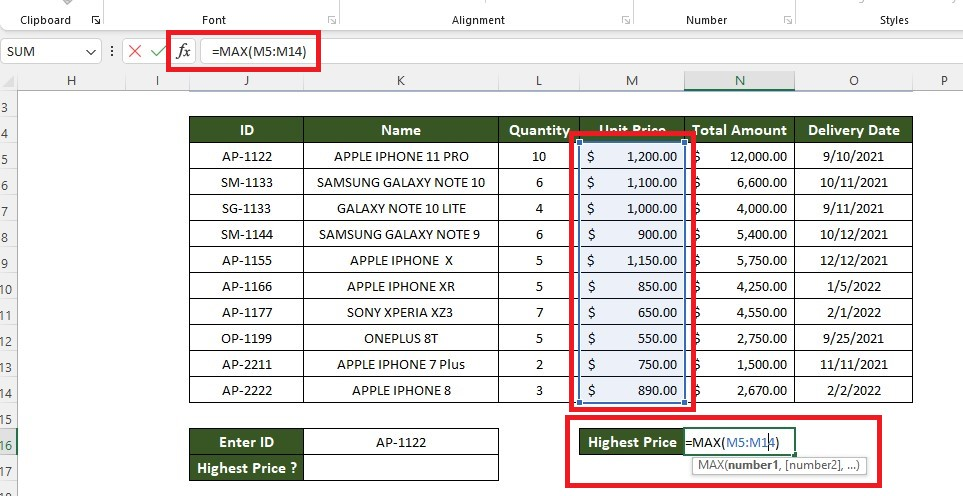1. Type the lookup value.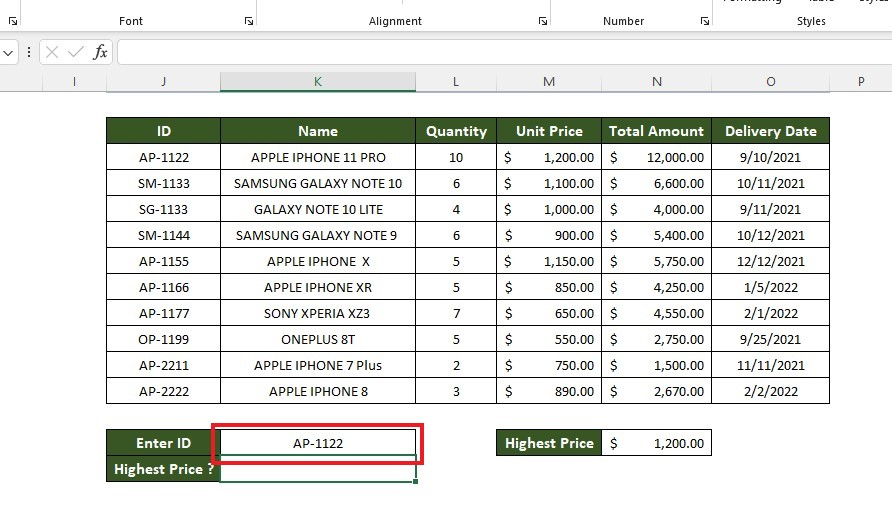1. Type the IF and VLOOKUP formula.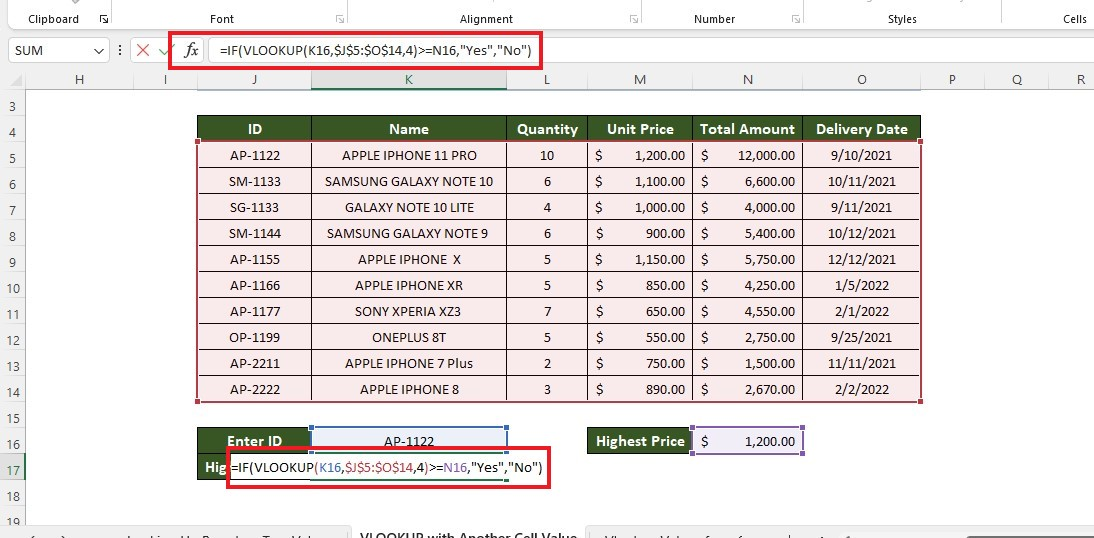1. Press the Enter key to get the IF and VLOOUP formula results with the MAX function value.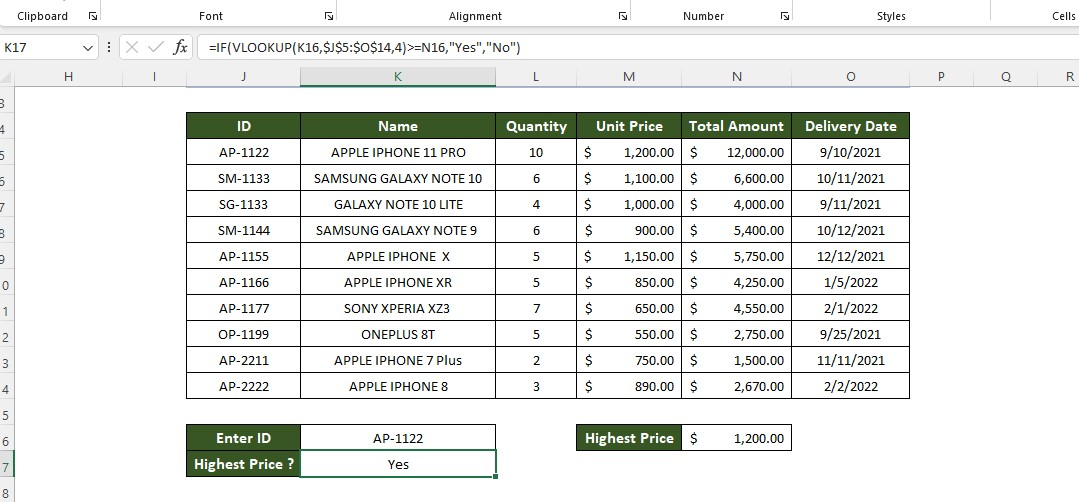Formula breakdown:

• We first check the condition using the VLOOKUP function's return value in the IF function. The VLOOKUP formula part will return the entered ID's price and be compared with the cell values.• If the entered ID's price is greater than or equal to the highest price, it will print Yes. Otherwise, it will generate No.

## Use Nested Function with IF & VLOOKUP to Lookup Values from a Shorter List

We must use nested functions IF and VLOOKUP to extract specific data from a list. A new column called "Status" has been added to the dataset.

The two possible values for this logical test are "Delivered" and "Not Delivered." Our job is to determine the status of each product using the information in the "Delivered Product List" table array.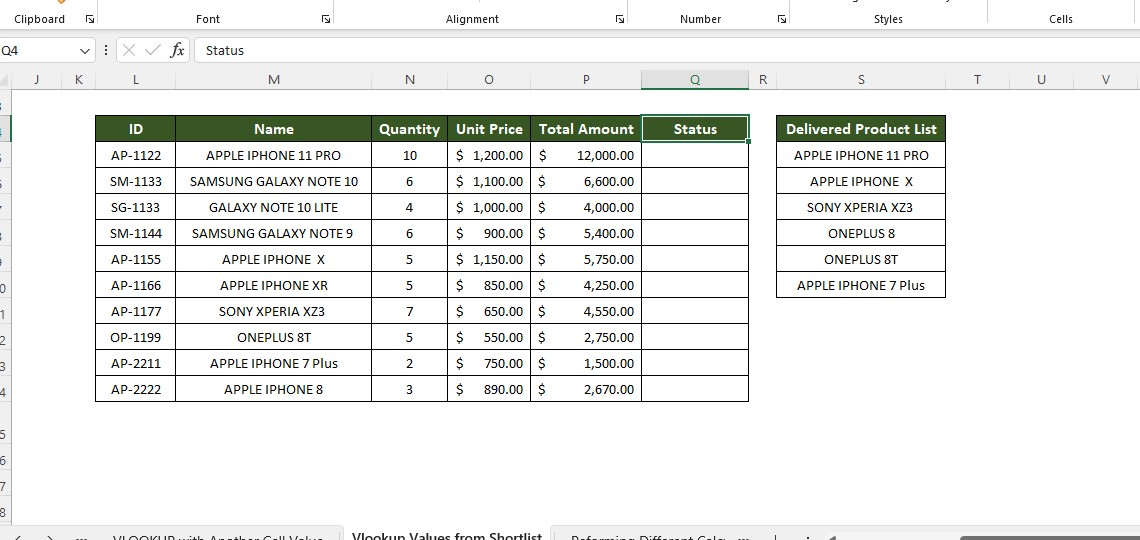To achieve this, follow the steps below.

1. Select a cell under the Status column.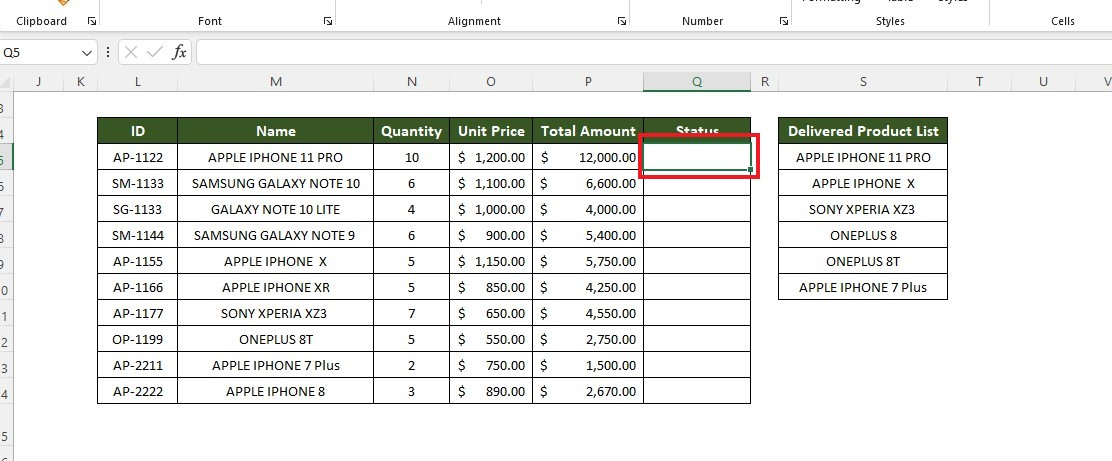1. Type and enter the formula.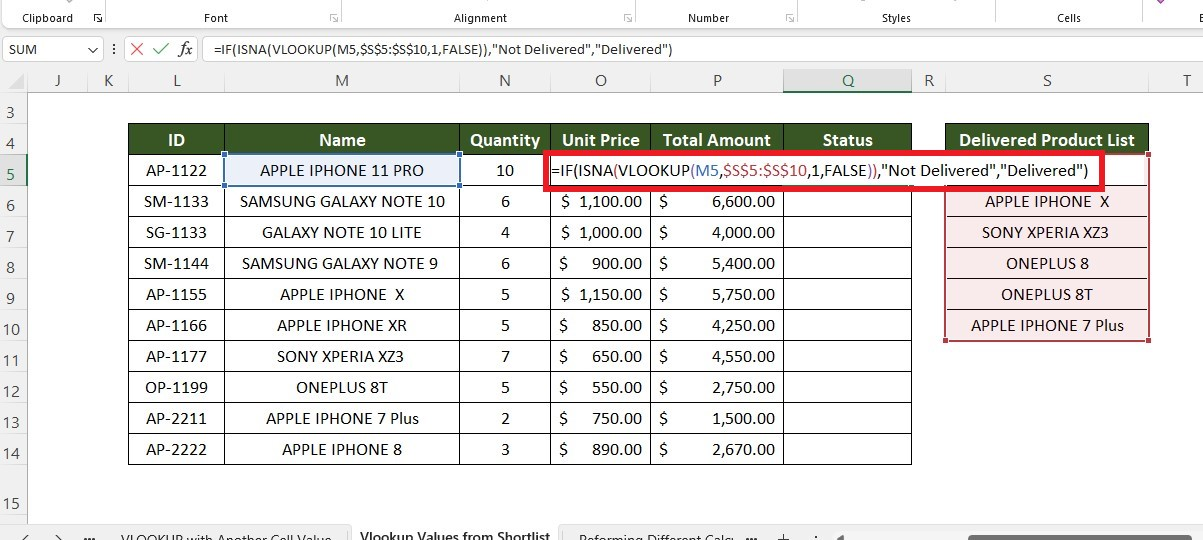1. Use the fill handle feature to fill the other rows.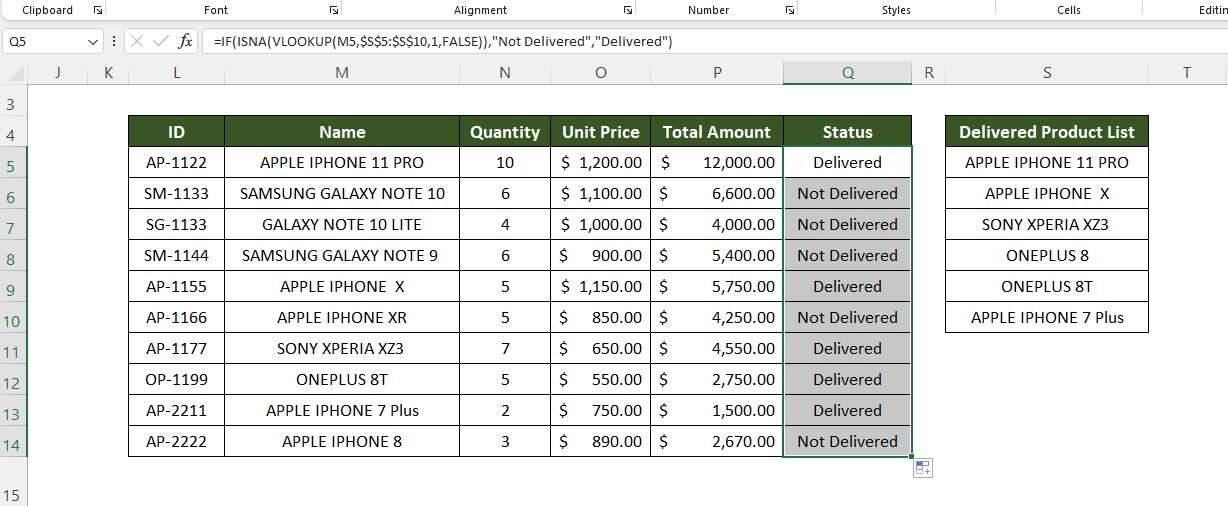Formula breakdown:

• We have used a combination of IF, ISNA, and VLOOKUP functions here.

• If the ISNA function cannot find the matched data name from the lookup range, it will return a Boolean value.

• Using the return value of the ISNA function, the IF function returns "Not Delivered" if the product is not found in the lookup range. Meanwhile, it will generate "Delivered" if the product is in the lookup table.

## Use of the IF and VLOOKUP Nested Function to Perform Different Calculations

Now, we will use the price of products to calculate discounts automatically. If the unit price is above \$800, we will calculate a 20% discount. Meanwhile, if the unit price is below \$800, we will calculate a 15% discount.

1. Type the product ID.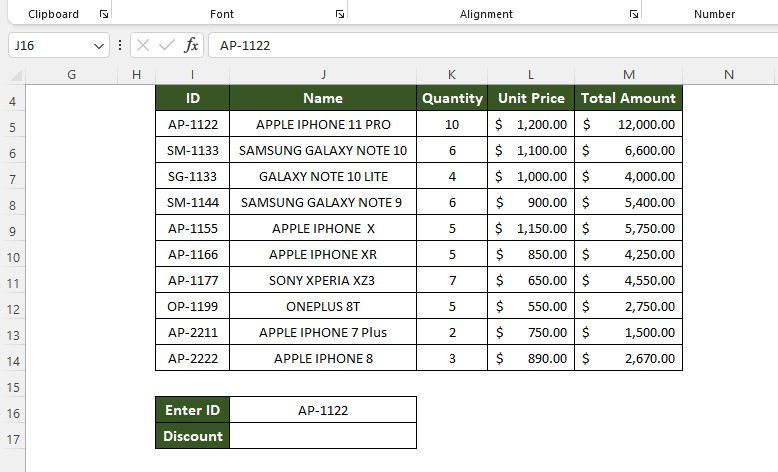1. Type the formula.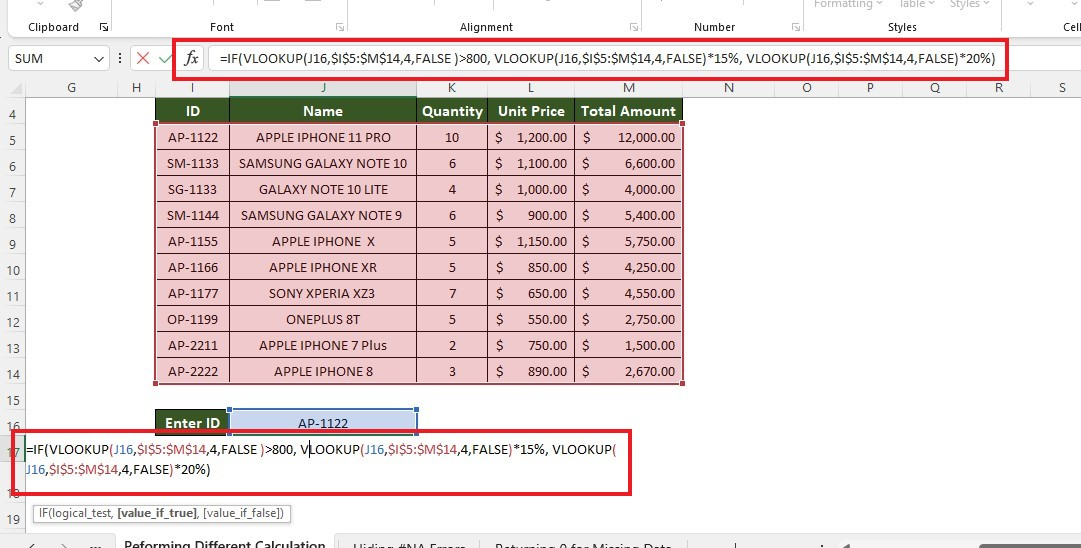1. Press the Enter key to get the result.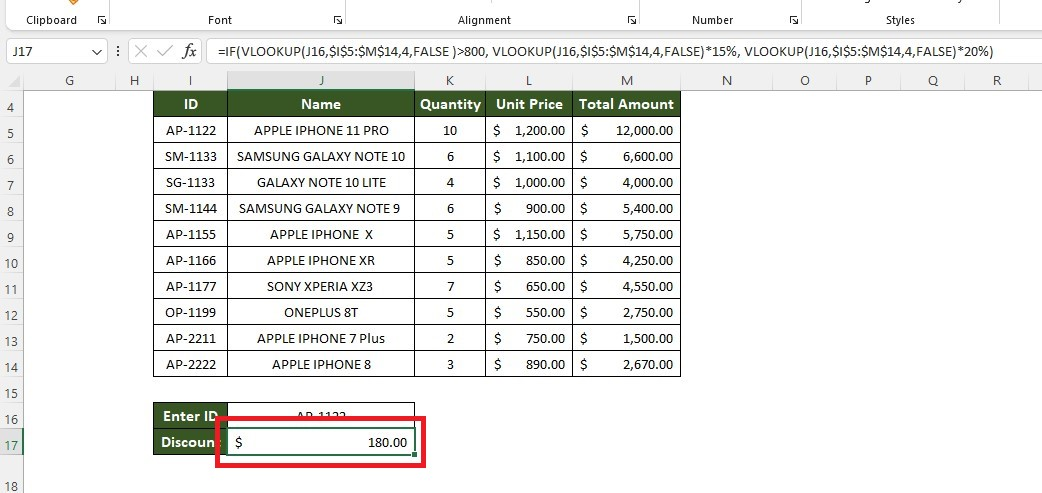Formula breakdown:

• The first VLOOKUP formula will check if the C16 cell's lookup value in the Unit Price column exceeds 800.• The whole formula ensures that if the lookup value exceeds 800, it will multiply by 15%. Otherwise, it will multiply by 20%.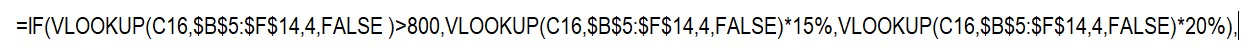## Final Thoughts on the IFS and VLOOKUP Nested Function

Overall, the IFS and VLOOKUP nested functions offer many features that can make navigating large datasets much easier.

The function can draw on multiple conditions and enable you to easily obtain what you need from the data with minimal user input.

Visit Simple Sheets for more easy-to-follow Excel guides and financial model examples, and remember to visit the related articles section of this blog post.

For the most straightforward Excel video tutorials, subscribe to Simple Sheets on YouTube!

## Frequently Asked Questions on the IFS and VLOOKUP Nested Function

### How many IF statements can I use?

Excel allows up to seven levels of nesting in its formulas for versions 2003 and older. Meanwhile, for versions 2007 and newer, you can nest up to 64 IF functions in a single formula.

### Why do I get an "#/N/A" error?

Here are the reasons:

• Wrong spelling lookup value

• Missing lookup value

• Incorrect table range

### Is there a function to avoid "#N/A" errors using the IF and VLOOKUP functions?

In Excel, it is common to use the combination of the ISNA function and the IF and VLOOKUP functions to manage and prevent #N/A errors.## Related Articles

What is a Sunburst Chart and When to Use a Sunburst Chart in Excel

How to Use SUMPRODUCT with Multiple Criteria in Excel

SUM Index-Match: What it is, and How do I use it?

#### Want to Make Excel Work for You? Try out 5 Amazing Excel Templates & 5 Unique Lessons

We hate SPAM. We will never sell your information, for any reason.